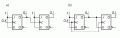# Counters,determining the next situation

#### avalox7

Joined Aug 9, 2020
31
I have a task to determine which sequence of situations the following counters are generating,the correct answers are for a) is serial counter which counts down the first condition in both counters are Q1Q0=0, this counter counts as 00,11,10,01,00.For b) the correct answer is synchronized counter which counts up:00,01,10,11,00.I want to know how this conditions are determined because I get different,from the truth table of JK.Also for a) I am a little bit confused because clock is connected to Q0,how will I express that when I am writing state equations?Here are the tasks https://prnt.sc/twz7kk.#### avalox7

Joined Aug 9, 2020
31
I have solved under b) I am just confused for a) when I write the truth table C1=Q0,how will that impact Q1 the following state?

#### RBR1317

Joined Nov 13, 2010
706
a) when I write the truth table C1=Q0
How will it help to write C1=Q0? The next state for Q1 is determined by its J&K inputs, and also by whether it receives a clock pulse (which is determined by the state of Q0 and its J&K inputs)?

#### avalox7

Joined Aug 9, 2020
31
How will it help to write C1=Q0? The next state for Q1 is determined by its J&K inputs, and also by whether it receives a clock pulse (which is determined by the state of Q0 and its J&K inputs)?